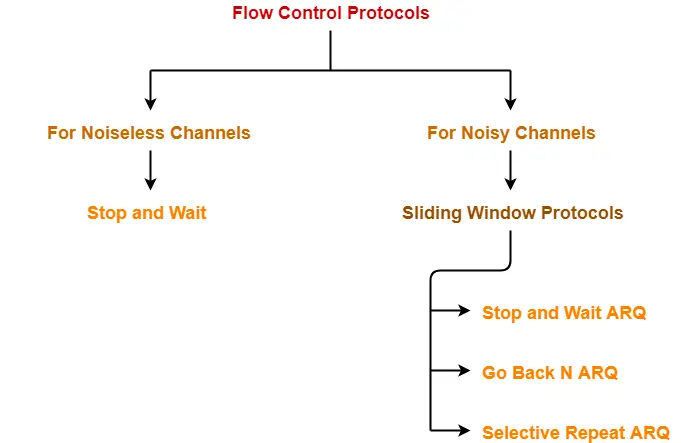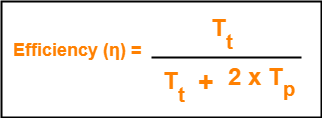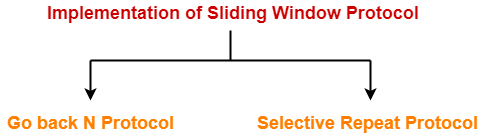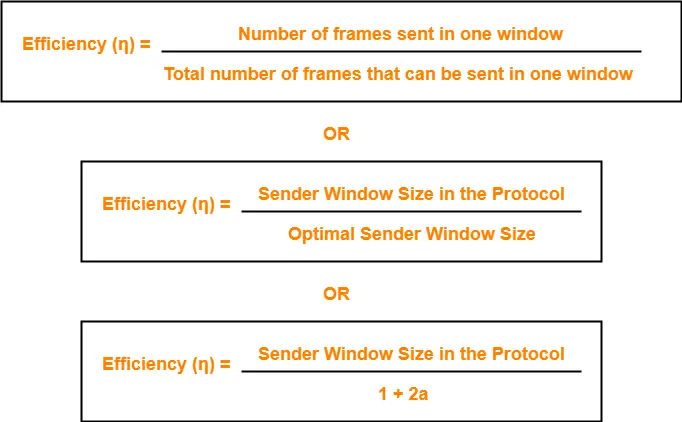# Sliding Window Protocol | Flow Control

## Flow Control Protocols-

In computer networking, there are various flow control protocols-## Sliding Window Protocol-

• Sliding window protocol is a flow control protocol.
• It allows the sender to send multiple frames before needing the acknowledgements.
• Sender slides its window on receiving the acknowledgements for the sent frames.
• This allows the sender to send more frames.
• It is called so because it involves sliding of sender’s window.

 Maximum number of frames that sender can send without acknowledgement = Sender window size

## Optimal Window Size-

 In a sliding window protocol, optimal sender window size = 1 + 2a

### Derivation-

We know,To get 100% efficiency, we must have-

η = 1

Tt / (Tt + 2Tp) = 1

Tt = Tt + 2Tp

Thus,

• To get 100% efficiency, transmission time must be Tt + 2Tp instead of Tt.
• This means sender must send the frames in waiting time too.
• Now, let us find the maximum number of frames that can be sent in time Tt + 2Tp.

We have-

• In time Tt, sender sends one frame.
• Thus, In time Tt + 2Tp, sender can send (Tt + 2Tp) / Tt frames i.e. 1+2a frames.

Thus, to achieve 100% efficiency, window size of the sender must be 1+2a.

## Required Sequence Numbers-

• Each sending frame has to be given a unique sequence number.
• Maximum number of frames that can be sent in a window = 1+2a.
• So, minimum number of sequence numbers required = 1+2a.

 To have 1+2a sequence numbers, Minimum number of bits required in sequence number field = ⌈log2(1+2a)⌉

## NOTE-

• When minimum number of bits is asked, we take the ceil.
• When maximum number of bits is asked, we take the floor.

## Choosing a Window Size-

The size of the sender’s window is bounded by-

• Receiver’s ability to process the data bounds the sender window size.
• If receiver can not process the data fast, sender has to slow down and not transmit the frames too fast.

### 2. Sequence Number Field-

• Number of bits available in the sequence number field also bounds the sender window size.
• If sequence number field contains n bits, then 2n sequence numbers are possible.
• Thus, maximum number of frames that can be sent in one window = 2n.

 For n bits in sequence number field, Sender Window Size = min (1+2a , 2n)

## Implementations of Sliding Window Protocol-

The two well known implementations of sliding window protocol are-## Efficiency-

Efficiency of any flow control protocol may be expressed as-### Example-

In Stop and Wait ARQ, sender window size = 1.

Thus,

Efficiency of Stop and Wait ARQ = 1 / 1+2a

## Problem-01:

If transmission delay and propagation delay in a sliding window protocol are 1 msec and 49.5 msec respectively, then-

1. What should be the sender window size to get the maximum efficiency?
2. What is the minimum number of bits required in the sequence number field?
3. If only 6 bits are reserved for sequence numbers, then what will be the efficiency?

## Solution-

Given-

• Transmission delay = 1 msec
• Propagation delay = 49.5 msec

### Part-01:

To get the maximum efficiency, sender window size

= 1 + 2a

= 1 + 2 x (Tp / Tt)

= 1 + 2 x (49.5 msec / 1 msec)

= 1 + 2 x 49.5

= 100

Thus,

For maximum efficiency, sender window size = 100

### Part-02:

Minimum number of bits required in the sequence number field

= ⌈log2(1+2a)⌉

= ⌈log2(100)⌉

= ⌈6.8⌉

= 7

Thus,

Minimum number of bits required in the sequence number field = 7

### Part-03:

If only 6 bits are reserved in the sequence number field, then-

Maximum sequence numbers possible = 26 = 64

Now,

Efficiency

= Sender window size in the protocol / Optimal sender window size

= 64 / 100

= 0.64

= 64%

## Problem-02:

If transmission delay and propagation delay in a sliding window protocol are 1 msec and 99.5 msec respectively, then-

1. What should be the sender window size to get the maximum efficiency?
2. What is the minimum number of bits required in the sequence number field?
3. If only 7 bits are reserved for sequence numbers, then what will be the efficiency?

## Solution-

Given-

• Transmission delay = 1 msec
• Propagation delay = 99.5 msec

### Part-01:

To get the maximum efficiency, sender window size

= 1 + 2a

= 1 + 2 x (Tp / Tt)

= 1 + 2 x (99.5 msec / 1 msec)

= 1 + 2 x 99.5

= 200

Thus,

For maximum efficiency, sender window size = 200

### Part-02:

Minimum number of bits required in the sequence number field

= ⌈log2(1+2a)⌉

= ⌈log2(200)⌉

= ⌈7.64⌉

= 8

Thus,

Minimum number of bits required in the sequence number field = 8

### Part-03:

If only 6 bits are reserved in the sequence number field, then-

Maximum sequence numbers possible = 27 = 128

Now,

Efficiency

= Sender window size in the protocol / Optimal sender window size

= 128 / 200

= 0.64

= 64%

To gain better understanding about sliding window protocol,

Watch this Video Lecture

Next Article- Practice Problems On Sliding Window Protocol

Get more notes and other study material of Computer Networks.

Watch video lectures by visiting our YouTube channel LearnVidFun.

SummaryArticle Name
Sliding Window Protocol | Flow Control
Description
Sliding Window Protocols in computer networks are the flow control protocols. Go Back N and Selective Repeat are the implementations of sliding window protocol. Sliding Window Protocol allows the sender to send multiple frames before needing acknowledgements.
Author
Publisher Name
Gate Vidyalay
Publisher Logo